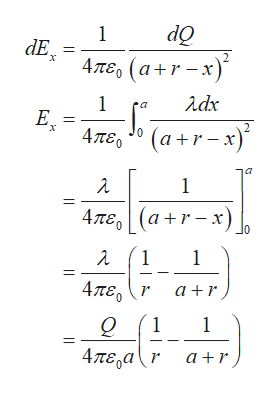# Positive charge Q is distributed uniformly along the x-axis from x=0 to x=a. A positive point charge q is located on the positive x-axis at x=a+r, a distance r to the right of the end of Q.Calculate the x-component of the electric field produced by the charge distribution Q at points on the positive x-axis where x>a.Express your answer in terms of the variables Q, a, r, and and appropriate constants. Calculate the y-component of the electric field produced by the charge distribution Q at points on the positive x-axis where x>a.Express your answer in terms of the variables Q, a, x, and appropriate constants. Calculate the magnitude of the force that the charge distribution Q exerts on q.Express your answer in terms of the variables Q, q, a, r, and appropriate constants. Calculate the direction of the force that the charge distribution Q exerts on q.

Question
3101 views

Positive charge Q is distributed uniformly along the x-axis from x=0 to x=a. A positive point charge q is located on the positive x-axis at x=a+r, a distance r to the right of the end of Q.

Calculate the x-component of the electric field produced by the charge distribution Q at points on the positive x-axis where x>a.
Express your answer in terms of the variables Q, a, r, and and appropriate constants.

Calculate the y-component of the electric field produced by the charge distribution Q at points on the positive x-axis where x>a.
Express your answer in terms of the variables Q, a, x, and appropriate constants.

Calculate the magnitude of the force that the charge distribution Q exerts on q.
Express your answer in terms of the variables Q, q, a, r, and appropriate constants.

Calculate the direction of the force that the charge distribution Q exerts on q.
check_circle

Step 1

The charge Q is uniformly distributed along the axis x = 0 to x = a. The linear charge density of the charge distribution is

Step 2

The charge across the small segment is

Step 3

The electric field strength due...help_outlineImage Transcriptioncloseδν Απε, (α+r- 3) 1 dE, 'Χ λάκ 1 Ε. х (а +r- х)" 2 (a rx) 4те, Ο λ 1 4πε, | (α +r- x). λ 1 1 4πε, r α+r 1 1 4πε,α (r α+r fullscreen

### Want to see the full answer?

See Solution

#### Want to see this answer and more?

Solutions are written by subject experts who are available 24/7. Questions are typically answered within 1 hour.*

See Solution
*Response times may vary by subject and question.
Tagged in